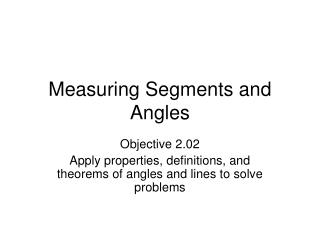DownloadDownload PresentationMeasuring Segments and Angles

# Measuring Segments and Angles

Télécharger la présentation## Measuring Segments and Angles

- - - - - - - - - - - - - - - - - - - - - - - - - - - E N D - - - - - - - - - - - - - - - - - - - - - - - - - - -
##### Presentation Transcript

1. Measuring Segments and Angles Objective 2.02 Apply properties, definitions, and theorems of angles and lines to solve problems

2. Warm-Up 1. 2. 3. 4. What is the complementary angle of 43 degrees? 5. What is the supplementary angle of 143 degrees?

3. Congruent line segments are simply segments with the same measure (length). If segment AB is congruent to segment CD, we write: AB CD In geometrical figures, two segments are shown to be congruent by marking them with the same number of small perpendicular marks, as shown below.

4. Find which two of the segments XY, ZY, and ZW are congruent. Because XY = ZW, XYZW.

5. If AB = 25, find the value of x. Then find AN and NB. AN = 10 and NB = 15, which checks because the sum of the segment lengths equals 25.

6. M is the midpoint of RT. Find RM, MT, and RT.

7. For every angle, there exists a line that divides the angle into two equal parts. This line is known as the angle bisector.

8. Find the measure of a

9. Find the value of x. x 30 x + 30 = 180 - 30 - 30 x = 150

10. Find the value of x x + 3x + 20 = 90 x 4x + 20 = 90 3x + 20 - 20 -20 4x = 70 4 4 x = 17.5

11. Homework: Worksheet Practice 1-4